# Analysis of Radar and Air-Visual UFO Observations on 24 October 1968 at Minot AFB, North Dakota, USA

Claude Poher, Ph.D.

## Part 4. 3-D Analysis of the Radarscope Photographs

### 4.1. B-52 Altitude and Radar Antenna Tilt-up

Let us now return to the calculations presented in equations (21) to (30), which determine fairly precisely the location of the B-52. We also have a good idea of its speed (254 knots), even though the calculations below were established for an average speed at 250 kts We also know that:

Dis783 = 35 - 18.8 nm = 16.2 nm         (32)

Dis783 - Dis771 = 2.57 nm. Therefore, Dis771 = 13.63 nm.         (33)

Let us assume that this distance is determined from the beginning of the descent at 35 nm from the TACAN transmitter:

Alt771 + 0.284 = 3.2625 - 0.1279048 Dis771.         (22)

Therefore:

Alt771 = 1.235 nm or 7500 feet         (34)

and we determine that:

Alt783 = 0.8272727 Alt771         (12)

Alt783 = 1.022 nm or 6205 feet.         (35)

These are ground altitudes and not mean sea level altitudes, which would be resolved by adding 1723 feet. As a result of these calculations, we have positioned the B-52 in the three axes during the sequence of the radarscope photographs. Let us now return to the determination of the tilt angle of the antenna radar and review our data thus far:

• Radius of the altitude hole in photo 771 = 2.20 nm

• Alt771 = ground altitude of the B-52 during photo 771 = 1.235 nm

• Radius of the altitude hole in photo 783 = 1.82 nm

• Alt783 = ground altitude of the B-52 during photo 783 = 1.022 nm.

We have now verified that the real altitude of the B-52 is less than the radius of the altitude hole in the photos. Next we will reprise the variables we used in prior equations:

• Tilt = tilt angle of the radar antenna towards the sky (tilt-up). Tilt is also the half angle at the summit of the blind cone of the radar, centered on the nadir of the B-52.

• K = Cos (Tilt)

• Alt771 = ground altitude of the B-52 during photo 771 (in nautical miles equivalent to 1 nm = 1852 m, or 6072 feet).

• Alt783 = ground altitude of the B-52 during photo 783 (in nautical miles).

Alt771 = 2.20 K         (1)

Alt783 = 1.82 K.         (2)

Therefore, with the values we generated in equations (34) and (35):

K = Alt771 / 2.20 = 0.5613636         (1 bis)

K = Alt783 / 1.82 = 0.5615385         (2 bis)

• Tilt = Arc Cos (K) = 55.8°.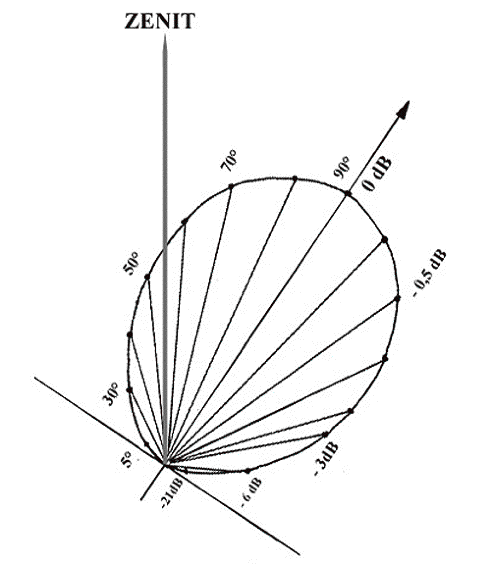Figure 43. This illustration presents the probable radar microwave beam at the calculated tilt (55.8°).

In effect, we know from the training manual that in Station Keep mode the radar could not capture information in the vertical direction below the aircraft, because the radar microwave beam (towards 9000 Mhz) was limited to about 60 degrees in the vertical plane. As a result of the previous calculations, 34.2 degrees downward, and 25.8 degrees above the horizontal. Of course, the angle between the nadir (vertical local downward) and the horizontal is equal to 90 degrees, and the radar would only begin receiving echoes in the downward direction of the ground at 45 degrees from the local vertical. It is therefore possible to carry out a trigonometric calculation to determine the altitude from the angular distance at which the radar begins to display the ground at the contrasted edge of the altitude hole. When we examine this transition of the radar return signal at the level of pixels we find: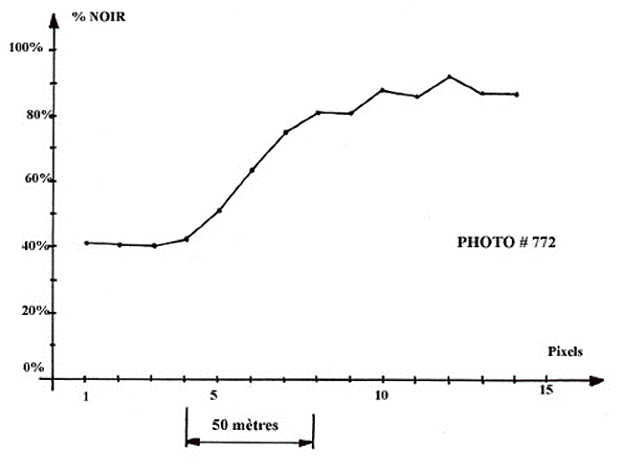Figure 44. Contrast transition of the radar signal at the edge of the altitude hole for photo 772 (all photos are identical). The complete transition is made in 4 to 5 pixels.

The transition is made over a distance of 50 meters, which is comparable to the resolution in distance of the radar stated in the technical manual. As a result, there was an inclination of 55.8 degrees of the radar antenna upwards with an abrupt reduction of the radar sensitivity towards the nadir.

It is interesting to note in Figure 44 that variations from pixel to pixel in the ground echo are the normal quantification noises of the radar and have an average value in the order of 80 to 90 %. The standard deviation of the fluctuations must be equal to the square root of the average value, which is 8.9 to 9.5 %, corresponding positively to the order of magnitude of the real fluctuations from pixel to pixel. This detail proves that these are actual radarscope photos, since the only way to acquire such a quantification noise is to receive a returned radar signal that is emitted in quanta (microwave photons). We can now return to the hypothesis of the position of the UFO beneath the B-52 and determine its parameters.

### 4.2. Hypothetical Displacements and Speeds of the UFO

We have considered that the UFO was probably located at a lower altitude than the B-52, except in photo 775. In these circumstances, the geometry for calculating distances is further complicated and we must take into account radar azimuths, as well as the values of the calculated alpha angles that are augmented by the 55.8-degree upward inclination of the antenna radar. This changes the traveled distances and the previous average speeds as follows:

• The position of the UFO changes between photos 771 and 772 from: 1.73 nm for 138° to 1.12 nm for 244°. Alpha + Tilt = 55.8 + 32 and 55.8 + 12.8° respectively. This indicates an angle nadir/UFO of 87.8° and 68.6°.

To solve this equation we must use spherical trigonometry. Let us call A the angle at the top of the triangle constituted by the segment B52-UFO of departure and the segment B52-UFO of arrival of the UFO, such that:

cos A = cos (68.6°) . cos (87.8°) + sin (68.6°) . sin (87.8°) . cos (244° - 138°)         (36)

Let us call D the distance traveled by the UFO:

D2 = (1.73)2 + (1.12)2 - 2 . 1.73 . 1.12 . cos A         (37)

• This corresponds to a displacement D = 2.277 nm in 3.77 seconds, which is an average speed of 2174 knots, or 4028 km / h (2502 mph).

It follows that:

• The position of the UFO changes between photos 772 and 773 from: 1.12 nm for 244° to 1.05 nm for 039°. Alpha + Tilt = 55.8 + 12.8 and 55.8 + 13.3° respectively. This indicates an angle nadir/ UFO of 68.6° and 69.1°. This corresponds to a displacement D = 1.98 nm in 1.29 seconds, which is an average speed of 5515 knots, or 1 0213 km / h (6346 mph).

• The position of the UFO changes between photos 773 and 775 from: 1.05 nm for 039° to 1.69 nm for 348°. Alpha + Tilt = 55.8 + 13.3 and 55.8 + 45° respectively. This indicates an angle nadir/ UFO of 69.1° and 100.8°. This corresponds to a displacement D = 1.47 nm in 5.58 seconds, which is an average speed of 945 knots, or 1750 km / h (1087 mph).

A possible interpretation of the 100.8 degrees for the angle nadir / UFO is that the UFO was slightly above the B-52 altitude during photo 775, if UFO and ground radar reflectivity were the same.

• The position of the UFO changes between photos 775 and 776 from: 1.69 nm for 348° to 1.08 nm for 040°. Alpha + Tilt = 55.8 + 45° and 55.8 + 12.8° respectively. This indicates an angle nadir/ UFO of 100.8° and 68.6°. This corresponds to a displacement D = 1.49 nm in 3.43 seconds, which is an average speed of 1563 knots, or 2894 km / h (1860 mph).

Note that the speeds are relative to the B-52’s forward speed at about 250 knots, or 460 km / h (285 mph). In fact, these calculations show only minor UFO position changes across photos.

### 4.3. Minimal Accelerations of the UFO

Since the UFO trajectory is angular, we might want to hypothesize a uniform acceleration of the UFO across photos, even though we do not know exactly how the UFO changed relative directions between photos. Nevertheless, we can calculate minimal accelerations of the UFO. If A is the acceleration, and D the distance traveled during time T, we will have the following relationship:

A = 2 . D / T2         (38)

• Between photos 771 and 772 the UFO position changes by 2.277 nm ( 4217 m) in 3.77 seconds, corresponding to an acceleration of 493 m/s 2 . This is more than 60 g .

• Between photos 772 and 773 the UFO position changes by 1.98 nm ( 3667m) in 1.29 seconds, corresponding to an acceleration of 4407 m/s 2. This is more than 449 g .

• Between photos 773 and 775 the UFO position changes by 1.47 nm ( 2722 m) in 5.58 seconds, corresponding to an acceleration of 175 m/s 2 . This is about 18 g .

• Between photos 775 and 776 the UFO position changes by 1.49 nm ( 2759 m) in 3.43 seconds, corresponding to an acceleration of 469 m/s 2 . This is about 48 g .

All of these accelerations are compatible with the performances necessary for an interstellar voyage.

Furthermore, during the course of the photo sequences:

• 771, 772, and 773, there is an abrupt change of direction and displacement of the UFO executed in less than 1.3 seconds, at a 50-degree angle towards the right.

• 772, 773, and 775, there is an abrupt change of direction and displacement of the UFO executed in less than 6 seconds, at a 50-degree angle towards the left.

• 773, 775, and 776, there is an abrupt change of direction and displacement of the UFO executed in less than 4 seconds, at an angle of 180 degrees.

Since the radarscope only displays a static transitory position of the UFO, at the brief and precise location when the radar beam swept it, we have no means to determine the radius of these turns, nor whether they were direct displacements followed by an abrupt stop and acceleration in a new direction (or whether there were multiple UFOs). Nevertheless, given that the radar echoes are instantaneous, it is reasonable to consider a scenario in which the echo was actually in constant motion throughout the series of photos.

### 4.4. Minimal Speeds Attained by the UFO

The hypothesis of a UFO located below the altitude of the B-52 for most of the photo sequence (except during the course of photo 775) is further reinforced by the variations of the intensity of the radar echo between photos. This hypothesis is also confirmed by the fact that photo 774 does not show an echo, which suggests a passage of the UFO beneath the B-52 in the blind cone of the radar. The summit angle of this cone is equal to 90 degrees. Based on the minimum UFO acceleration speed A at time T we can calculate speed V as follows:

V = A T         (39)

• Between photos 771 and 772 acceleration is 493 m/s 2 during 3.77 seconds; the speed could be 1858 m/s (Mach 5.4). That is 6688 km / h (4155 mph).

• Between photos 772 and 773 acceleration is 4407 m/s 2 during 1.29 seconds; the speed could be 5685 m/s (Mach 16.7). That is 20466 km / h (12716 mph).

• Between photos 773 and 775 acceleration is 175 m/s 2 during 5.58 seconds; the speed could be 977 m/s (Mach 2.9). That is 3515 km / h (2184 mph).

• Between photos 775 and 776 acceleration is 469 m/s 2 during 3.43 seconds; the speed could be 1609 m/s (Mach 4.7). That is 5791 km / h (3598 mph).

These calculations show that the UFO was supersonic during its flight, which should have produced a loud sonic boom time and again, though none was reported, as is generally the case with other reported supersonic UFOs. Nevertheless, these speeds are clearly beyond all aeronautical developments in 1968.

### 4.5. 3-D Positions of the UFO in Relation to the B-52

In Figure 45, I have illustrated the angular distances of the UFO in relation to the B-52 for photos 771, 772, 773 and 775, based on the indicated azimuth direction, and calculated altitude angle in the respective radarscope photos.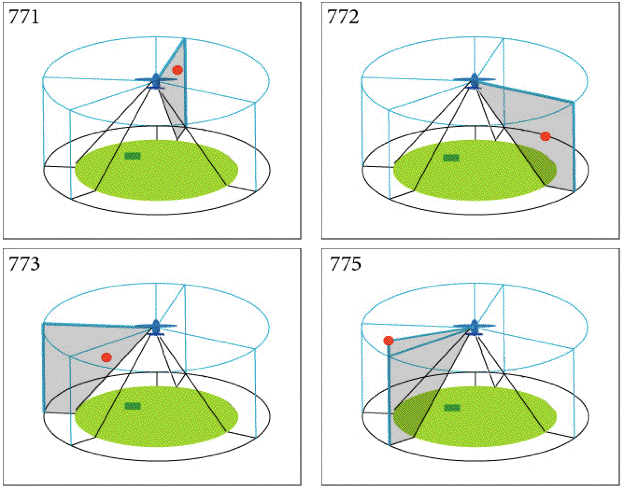Figure 45. Sequence of four illustrations showing UFO positions in relation to the B-52. For photos 776-782 the position of the UFO is consistent with 773 at roughly 1 nmi off the left wing.

The external circle has a radius of 4 kilometers, and the successive positions of the UFO (red circle) in relation to the B-52 are roughly to this scale in distance. However, the ground altitude, and sizes of the UFO and B-52 are not to scale. The solid green circle represents the blind radar zone, extending from the B-52 to the ground beneath the B-52, and the small green rectangle represents an imaginary detail of the landscape under the aircraft. The successive azimuth directions (vertical planes) are shaded and highlighted in bold blue. Although this illustration is imprecise, it will help to visualize the trajectory of the UFO in 3-dimensional space.

The illustration in the lower right represents radarscope photo 775, in which the UFO is located above the altitude of the B-52. This position takes into account two facts. On the one hand, the UFO echo is as intense as the ground echo on this photo, so it is conceivable that the UFO was closer to the axis of the radiance lobe of the radar antenna and therefore above the horizontal plane. On the other hand, it is necessary to take into account the successive intensities of the radar echo of the UFO, as well as the radiance diagram of the radar antenna in the vertical plane. Let us review the results of the photometric measurements on the radarscope photos: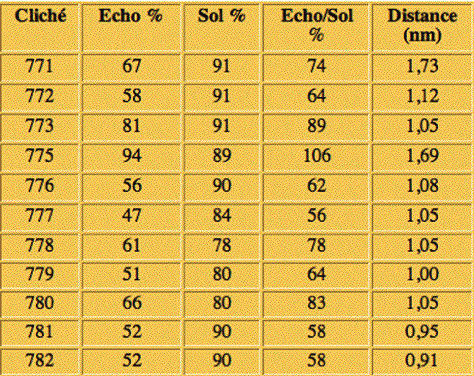Table 6. Results of the photometric study of the radar photos. Sol = Ground.

In Table 6, the third column (Echo/Sol%) presents the ratio of the intensity of the UFO echo, divided by the intensity of the ground echo at the edge of the altitude hole. We see that during photo 775 the echo has about the same intensity as the ground, and the distance separating the UFO from the B-52 is 1.69 nm (3130 meters). Earlier, we calculated the ground altitude of the aircraft for photos 771 and 783, and found:

• Alt771 = 7500 feet ( 1.235 nm, or 2288 m)

• Alt783 = 6205 feet ( 1.022 nm, or 1893 m).

During photo 775 the distance between the UFO and the B-52 is greater than the distance between the B-52 and the ground. Therefore, if the radar albedo of the UFO and the ground are assumed to be of the same magnitude, and the sensitivity is equal in both directions, we should receive radar signals of different intensities. However, we do not accurately know the sensitivity of the radar versus the direction of the target in the vertical plane, although the technical training manual indicates that it varies in cosecant square. Since we have calculated the tilt angle of the antenna upwards at 55.8 degrees, the sensitivity of the B-52 radar in the vertical plane probably varies as the theoretical function represented in Figure 46: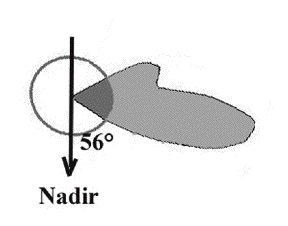Figure 46. Diagram of vertical sensitivity of the B-52 radar antenna tilted up by 55.8°.

The radar antenna rotates around a vertical axis with a maximum sensitivity at roughly 10 degrees under the horizon. It is therefore blind within an angle of 111.6 degrees below the B-52 as confirmed by the photographs. We also note that the ground echo at the periphery of the blind zone corresponds to a point of low radar sensitivity, which would attenuate to 20 dB in comparison to the maximum sensitivity. Since the intensity of the radar echo of the UFO is of the same magnitude as the intensity of the ground echo at 56 degrees from the vertical, and at a ground altitude of the same distance of the UFO, this would mean that the signal of the UFO echo was attenuated even more and by different causes than the signal of the ground echo. Because the ground echo is 1.4 times the distance separating the UFO from the B-52, in principle, the ground echo was more distance-attenuated by a factor of 1.44 = 3.8 or 6 dB. Therefore, the UFO echo should be two times brighter than the ground echo for the same albedo, which is not the case.

Two possible causes exist for this difference in attenuation: a different orientation in the radar antenna diagram, or some absorption inside the ion cloud surrounding the UFO. In order to reconcile photometric results with the established sensitivities of the radar in the vertical plane, we have assumed that during photo 775 the UFO was essentially at the same altitude as the airplane, and possibly a little above it. This assumption has to remain conjecture because we cannot infer the exact sensitivity diagram of the B-52 radar antenna, and the absorption of the ion cloud. However, by comparing the successive photos and assuming a constant ionic absorption, the hypothesis that the UFO was higher in altitude than the B-52 during photo 775 seems the most plausible solution given the physical evidence. Note that in photo 774 the echo of the UFO has disappeared, which would be possible if the UFO were situated in the blind cone of the radar beneath the B-52, resulting in a continuous UFO trajectory between photos 773 and 775.

The 3D reconstruction of the trajectory implies that the UFO spiraled below and around behind the B-52 at an extremely high rate of speed (in about 15 seconds), while also maintaining a relative distance of 1-2 nautical miles and the corresponding forward trajectory of the B-52 at 250 knots.

### 4.6. Energetic Aspects of the UFO

As we have determined previously, the radar photographs show a very rapid displacement of the UFO as follows:

• Between photos 771 and 772, the UFO acceleration is 493 m/s 2 during 3.77 seconds, resulting in a speed of 1858 m/s (4155 mph).

• Between photos 772 and 773, the UFO acceleration is 4407 m/s 2 during 1.29 seconds, resulting in a speed of 5685 m/s (12176 mph).

• Between photos 773 and 775, the UFO acceleration is 175 m/s 2 during 5.58 seconds, resulting in a speed of 977 m/s (2184 mph).

• Between photos 775 and 776, the UFO acceleration is 469 m/s 2 during 3.43 seconds, resulting in a speed of 1609 m/s (3598 mph).

Furthermore, the ground witnesses described large angular speeds and some form of angular trajectories, which imply large accelerations. We will therefore calculate a magnitude of the minimal kinetic energy of the UFO during its rapid maneuvers. This parameter is of particular interest since it must conform to the natural law of energy conservation, that is, the kinetic energy of a moving mass must be derived from somewhere. The only available information we have comes from terrestrial technology, which suggests that the energy must come from the aircraft’s engine and its onboard fuel supply. The calculation of the kinetic energy Ec relies on a well known classical mechanics law, where the mass M of the craft, and its speed V are related as follows:

• Ec = ½ M V 2 .

Note that we are conservative in our estimation by ignoring the total mass M of the observed UFO. We shall postulate several hypotheses given our empirical data. Firstly, that the UFO (or UFOs) might have been an inhabited vehicle because the trajectory was seemingly strategic and the vehicle was sizeable and traveled at an enormous speed.

In 1977, I assembled the dimensions and total masses of various kinds of terrestrial vehicles in order to get comparative references to determine their average statistical mass per cubic meter. In this instance, if we know the size of a UFO, we can calculate its volume and these statistics could help us determine its approximate total mass by anthropomorphic hypotheses. The 1977 statistics revealed an unexpected result. The dispersion of the density of all types of vehicles is relatively low, and this density is almost statistically always between two extremes that are separated by a factor of only two.

At one end are heavy technology vehicles such as trains, trucks, commercial aircraft and competition sail boats, which are around 300 kg / m 3. At the other end are light technology vehicles such as private aircraft, cars, automatic satellites and space stations, which are around 150 kg / m 3. Based on these two extremes, we shall assume that the total mass of the Minot UFO was between 150 and 300 kg per cubic meter of the total volume. The dimensions of the Minot UFO have been deduced from the various witness descriptions and are fairly coherent. Of course, the following considerations are not accurate values but orders of magnitude. For our evaluation we will use the UFO dimensions reported by the B-52 copilot Capt. Runyon, since he observed the UFO in close proximity.

From Figure 56 we determine that the UFO had a complex volume of about 60 x 30 x 15 meters, which is a total volume in the order of 27000 cubic meters. Two of the ground witnesses reported that the UFO was “slender” and had a smaller height than the horizontal width compared to the UFO reported by the pilots. If the UFO shape were as flat, as is suggested by the drawings, the total volume would be about half to a third of the previous figure, which would simply mean that we have to divide our final results by a factor of 2 or 3. In any case, these adjustments would not change the conclusion. According to the average statistical densities of terrestrial vehicles, the Minot UFO might have had a total mass between 4 and 8 million kg (4000 to 8000 metric tons). Consequently, by using the previous equation to calculate the kinetic energy Ec, we get:

• Between photos 771 and 772, the UFO acceleration is 493 m/s 2 during 3.77 seconds, resulting in a speed of 1858 m/s; and a kinetic energy between 0.69 and 1.38 . 10 13 Joules.

• Between photos 772 and 773, the UFO acceleration is 4407 m/s 2 during 1.29 seconds, resulting in a speed of 5685 m/s; and a kinetic energy between 6.5 and 12.9 . 10 13 Joules.

• Between photos 773 and 775, the UFO acceleration is 175 m/s 2 during 5.58 seconds, resulting in a speed of 977 m/s; and a kinetic energy between 0.19 and 0.38 . 10 13 Joules.

• Between photos 775 and 776, the UFO acceleration is 469 m/s 2 during 3.43 seconds, resulting in a speed of 1609 m/s; and a kinetic energy between 0.52 and 1.03 . 10 13 Joules.

Thanks to the radarscope clock, we know the precise time in seconds between each photo during which the kinetic energy of the UFO was accumulated. Based on these data we can calculate the power output of the UFO engine. The power is simply the kinetic energy per second of time.

• Between photos 771 and 772, the UFO acceleration is 493 m/s 2 during 3.77 seconds, resulting in a speed of 1858 m/s; and a kinetic energy between 0.69 and 1.38 . 10 13 Joules. This corresponds to 1800 to 3600 gigawatts of kinetic energy per second of time.

• Between photos 772 and 773, the UFO acceleration is 4407 m/s 2 during 1.29 seconds, resulting in a speed of 5685 m/s; and a kinetic energy between 6.5 and 12.9 . 10 13 Joules. This corresponds to 50400 to 100800 gigawatts of kinetic energy per second of time.

• Between photos 773 and 775, the UFO acceleration is 175 m/s 2 during 5.58 seconds, resulting in a speed of 977 m/s; and a kinetic energy between 0.19 and 0.38 . 10 13 Joules. This corresponds to 340 to 680 gigawatts of kinetic energy per second of time.

• Between photos 775 and 776, the UFO acceleration is 469 m/s 2 during 3.43 seconds, resulting in a speed of 1609 m/s; and a kinetic energy between 0.52 and 1.03 . 10 13 Joules. This corresponds to 1500 to 3000 gigawatts of kinetic energy per second of time.

These powers are enormous. By comparison, a single modern nuclear electric power plant delivers a peak power of 1.3 gigawatts. Even if we simply consider the lowest values, the Minot UFO was capable of producing a mechanical power comparable to 260 to 39000 actual nuclear power plants. These enormous mean values make it unnecessary to calculate the maximum values.

Of course, these evaluations are approximations, but they are nevertheless justified given the reported and observed performances of the UFO confirmed by the B-52 radar. This magnitude of power demonstrated by a machine that has little in common with our current understanding of energy technology and production raises several fundamental questions:

• What is the nature of the UFO’s energy ? We know of nothing that could approach the preceding values.

• What is the source of the UFO’s energy ? We do not know of any means to supply such levels of energy and deliver it so quickly.

The order of magnitude of the acceleration allows us to extrapolate that this UFO would be able to attain a relativistic speed in a brief period of time (less than a day), if it could sustain the accelerations that were calculated. For example, acceleration in the order of the 450 g’s observed between photos 772 and 773 would attain 80% of the speed of light in approximately 15 hours. Given this speed, the relativistic time compression would become negligible, and the onboard clock, as well as the metabolism of the occupants would slow down. Under such conditions, interstellar travel becomes compatible with the life expectancy of the occupants of the vehicle.

In order to declare with relative certainty that interstellar travel is feasible, we have to concede with difficulties other than speed. The second substantial difficulty is the massive quantity of kinetic energy necessary to attain a relativistic speed. This enormous energy must be expended to accelerate and decelerate, in order to arrive with a null speed. The Minot UFO suggests that in nature there exists a form of energy to accomplish this.

In any case, it seems to me that the discovery of this kind of energy and the means to extract it should be the highest priority for humankind, even if the Minot UFO observations bring only concordant clues and not absolute proofs.

### 4.7. Discussion 1: The B-52 Altitude and the Tilt-up Angle of the Radar Antenna

During the process of reconstructing the flight path of the B-52 and dynamic behavior of the UFO, we measured the following quantities from the radarscope photos:

• The B-52 oblique distance and azimuth to the Lake Darling feature in photo 783.

• The B-52 horizontal speed during photos 782 and 783.

• The UFO radar echo distance and azimuth in all photos where a UFO was painted.

• The UFO radar echo average speed in 2-D space during all photos where a UFO was painted.

• The 2-D radar size of the UFO target in the successive photos.

• Details have also revealed that the photos were made from a real aircraft having the characteristics of a B-52.

These measurements are quite consistent with the observer accounts, primary documentation and quantitative evidence. The unusual behavior of the UFO was clear in this reconstruction, particularly from the 2-D speeds and accelerations obtained during this first, more classical approach. These parameters are quite reliable because they were obtained from measurements made by a then state-of-the-art instrument. Notably, this type of radar is not able to provide 3-D (altitude) positions. However, we have several circumstances that allow us to theoretically determine the 3-D parameters from calculations based on known laws of physics and aeronautical constraints of the B-52 in flight. From these theoretical calculations we get the following parameters:

• The Tilt-up angle of the radar antenna.

• The B-52 altitude during the photos.

• The 3-D positions of the UFO.

• The 3-D minimum speeds of the UFO.

• The 3-D minimum accelerations of the UFO.

• The minimum kinetic energy of the UFO.

• The minimum power of the UFO propulsion.

• The approximate size of the cloud of ionized air surrounding the UFO, and the approximate theoretical minimum value of the particle charge density of this cloud.

• A theoretically coherent explanation for the VHF radio transmission failure.

For most of these theoretical calculations the necessary assumptions are known, but certain calculations contain hidden hypotheses that should be discussed in order to provide an understanding of the limits of the methods used. In order to grasp these hidden hypotheses, it is necessary to recall that the photos of the B-52 radarscope were taken during the descent trajectory from the WT fix. The potential trajectories were represented in Figure 47 (below) at an altitude scale five times that of the distance scale.

Here we face the first difficulty: In order to calculate the B-52 altitude during photo 783 (a position corroborated by the radar image of Lake Darling), we need a map to ascertain the B-52 distance from the Deering TACAN transmitter adjacent to the runway. Our original calculations were made with a map of the region that was included in the Blue Book documents, which was not at the same scale as the map used by Werlich in 1968.

In his research of the Minot events, Tulien realized several anomalies concerning the position of the WT fix on the original map, and located a more accurate USGS map that covers the entire B-52 descent trajectory. Although the alignment of Col. Werlich’s overlay did not match the USGS map, he had drawn several auxiliary details on his overlay, including contours of several small cities and roads, six latitude and longitude coordinates, and the virtual position of the initial approach fix, referred to as the “WT fix.” After diagnosing the problems, we were able to adjust the overlay so that all of the details were in alignment on the USGS map, including the WT fix position, which was finally known with accuracy of about 35 meters (or about the same as the B-52 radar resolution). We discovered a different distance from the B-52 position during photo 783 to the Deering TACAN, which required a re-calculation of all the values we had originally obtained.

Here we found a second difficulty. Two of the first parameters calculated from the B-52 position during photo 783 were the B-52 altitude, and the radar antenna tilt-up angle, which correspond to the diameter of the altitude hole on each photo.

The original map resolved a tilt-up angle of 45 degrees, which was consistent with the indications of the technical training manual for the radar. However, we found a larger angle of 55.8 degrees on the USGS map, which does not seem compatible with radar system specifications in the technical training manual. As can be seen in Figure 47, the B-52 altitude is calculated from the vertical trajectory of the B-52 beginning at the WT fix (FL200) and ending at the runway. In order to present the illustration, we used a different yet more accurate scale for the distance and the altitude. The altitude scale is five times the distance scale--in which case the altitudes are exaggerated by a factor of five. Additionally, we specify the positions of the B-52 nadir during photos 771 and 783, and four possible trajectories from the WT fix to the runway.

We have a verification of the hidden hypothesis that the B-52 trajectory from the WT fix to the runway was a straight line because the radar position of the B-52 during photo 783 is perfectly aligned with the runway on the new map. There is an upper black trajectory called “Minimum slope” that is directly joining the WT fix with the west end of the runway. Next, there is a (red) trajectory called “Probable slope” that joins the WT fix with the Middle Marker (MM, or Final Approach Fix) where, according to Minot AFB approach procedures, the altitude of the B-52 would be 3200 feet MSL.

The existence of the MM beacon and the corresponding approach procedure is another hidden hypothesis because this information was obtained from current 2003 Instrument Approach Procedures (approach plates) for Minot AFB. However, the Instrument Landing System (ILS), which incorporates the MM radio beacon existed at most airports at the time, so it seems probable that the same 2003 ILS approach procedures were in effect in 1968.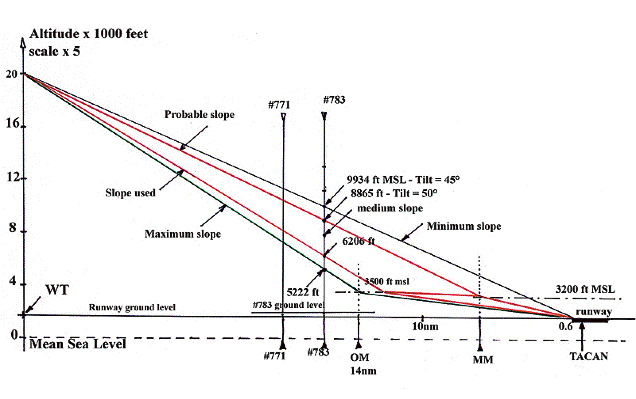Figure 47. Four possible vertical trajectories of the B-52 during the descent from the WT fix at 20,000 feet altitude to the runway at Minot AFB (1723 feet above MSL). The positions of photos 771 and 783 are indicated, including the corresponding altitudes for photo 783.

Next, there is a lower green “Maximum slope” that joins the WT fix with the Outer Marker (OM) situated 14 nautical miles from the TACAN, where the aircraft would be at altitude 3500 feet MSL. From OM the most probable trajectory would have been to MM at 3200 feet before descent to the runway. There are new hidden hypotheses associated with this “Maximum slope” trajectory.

Between the “Probable slope” and the “Maximum slope” hypothetical trajectories, there is another red trajectory (“Slope used,” or “Report slope”) descending to 3200 feet MSL, and leveling out to the MM before descending to the runway. This intermediate lower trajectory is a result of the simple recalculation of the parameters after changing the map scale for the position of the B-52 during photo 783.

Figure 47 also shows the different possibilities for the B-52 altitude that correspond to the different hidden hypotheses concerning the four B-52 descent trajectories.

We get the following altitude results:

• B-52 altitude during photo 783 = 9934 feet MSL for the “Minimum slope” hypothesis.

• B-52 altitude during photo 783 = 8865 feet MSL for the “Probable slope” hypothesis.

• B-52 altitude during photo 783 = 6206 feet MSL for the recalculated “Report slope” hypothesis.

• B-52 altitude during photo 783 = 5222 feet MSL for the “Maximum slope” hypothesis.

At each altitude the trigonometric equation generates a different value for the tilt-up angle of the radar antenna.

• A tilt-up angle of 45° for the “Minimum slope” hypothesis

• A tilt-up angle of 50° for the “Probable slope” hypothesis, etc.

Using this calculation, the 45-degree tilt-up angle seems to correspond to an impossible altitude for the B-52 at this moment. What is clear from the previous results is that the B-52 altitude varies by a factor of 1.9 when we take into account the hidden hypotheses. From the aeronautical (i.e., the pilot’s) point of view, it seems that the correct hypothesis is the descent that ends at the MM, although from the existing data we have no way to corroborate this hypothesis. This means that there is an uncertainty regarding the true B-52 altitude during photo 783. Further, because the tilt-up angle of the B-52 radar antenna is tied to the altitude hole radius on photo 783, we cannot determine the tilt-up angle that is used in all 3-D calculations of the UFO positions through its sine and cosine values:

• B-52 ground altitude = Photo alt hole radius. Cos (tilt up angle).

This means that the hidden hypothesis concerning the descent trajectory has a direct influence on the 3-D results. However, this influence is not substantial, because the trigonometric results do not change considerably when the angle of the radar antenna is modified by 10 to 15%. Nevertheless, we need to consider the question of the radar antenna tilt-up angle.

Let us suppose that the B-52 radar antenna reflector is almost flat in the vertical direction and has a parabolic shape only in the horizontal direction. This is the case with many mapping radars antennas, and such a reflector shape is shown in the sensitivity diagram of Figure 48.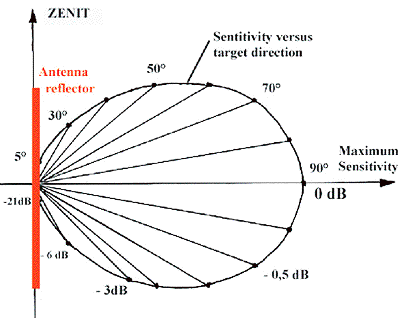Figure 48. Hypothetical radar antenna diagram in the vertical plane.

In such a diagram, the output microwaves flux Φ in a direction making an angle α with the nadir of the antenna, is simply given by:

Φ = Φmax sin (α)         (40)

where Φmax is the maximum flux emitted in the direction α = 90°. When such an antenna is tilted up 45 degrees we get the following hypothetical radar antenna diagram: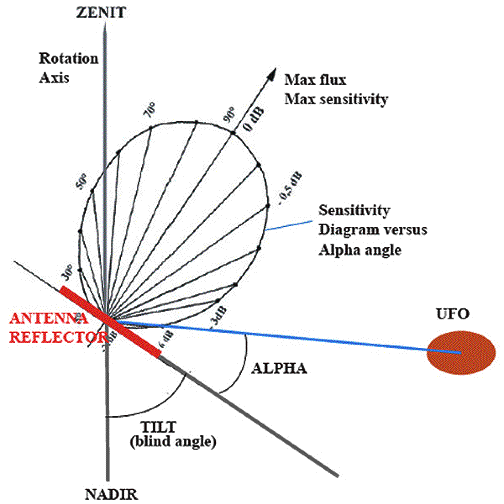Figure 49. Hypothetical radar antenna diagram when the antenna is tilted up 45 degrees. The hypothetical tilted up antenna has a “blind” cone in the direction of the nadir, and the summit angle of the blind cone is two times the tilt-up angle of the antenna.

Even though the radar antenna diagrams are hypothetical, we can nevertheless attempt to verify these diagrams with the existing data.Figure 50. Position of the B-52 during photos 771 and 783. The USGS map gird is in statute miles.

In order to understand our method of verification, let us first examine the scenario beneath the B-52 during photo 783, perpendicular to the B-52 trajectory in the direction towards the southwest (lower left). In Figure 50, the isocontours indicating a difference of 10 meters of altitude on the ground are quite distant from each other, which means that the ground is essentially flat along the 5 miles of the radar range. Now let us consider the vertical plane perpendicular to the B-52 trajectory, as seen from the rear of the aircraft. In Figure 51 we have reproduced the different altitudes of the B-52 from the different hidden hypotheses in Figure 47, except that in this instance we have the same vertical and horizontal scales. Five radar target directions are represented for the “Probable slope” trajectory. The length of the inclined lines, which join the position of the B-52 at 8865 feet MSL above the ground, measure exactly 5, 4, 3, and 2.5 nm. Finally, the altitude hole radius is a little less than 2 nm. The inclined altitude hole range hits the ground at a distance from the B-52 nadir that is not equal to the altitude hole radius of about 1.9 nm, but much closer at about 1.5 nm from the nadir. From this figure we can directly measure the tilt-up angle of the radar antenna:

• A Tilt-up angle of 50° for the “Probable slope” trajectory

• A Tilt-up angle of 45° for the “Minimum slope” trajectory

• A Tilt-up angle of 55.8° for the “Report slope” trajectory

• A Tilt-up angle of 71° for the “Maximum slope” trajectory.Figure 51. Vertical radar situation during photo 783. The B-52 altitude, and horizontal distance from the B-52 nadir are drawn at the same scale.

Thus, the tilt-up angle changes considerably depending on the possible B-52 trajectory hidden hypotheses. These hypotheses predict a possible trajectory for the B-52 from a theoretical point of view, and the “Probable slope” trajectory is based on aeronautical procedures that are plausible from a pilot’s point of view.

In Figure 51 the value of the α target angle is indicated for the different radar ranges for the probable trajectory hidden hypothesis:

• Angle α = 10° for a 2.5 nm range.

• Angle α = 15° for a 3 nm range.

• Angle α = 21° for a 4 nm range.

• Angle α = 25° for a 5 nm range.

There is another set of angles indicated in Figure 51, namely the incidence angle φ of the radar microwaves on the ground for different radar ranges:

• Angle φ = 29° for a 2.5 nm range.

• Angle φ = 24° for a 3 nm range.

• Angle φ = 18° for a 4 nm range.

• Angle φ = 14° for a 5 nm range.

This angle φ is significant regarding the microwave flux Φret returned by the ground towards the radar, because this returned microwave flux varies as a function of the Φin incoming flux:

Φret = Φin sin2 (φ)         (41)

The incoming flux is precisely the one defined by equation (40), divided by the square of the distance D:

Φin = Φmax sin (φ) / D2         (42)

The echo flux Φecho producing the radar signal painted on the radar screen is simply the returned flux Φret divided by the square of the distance D:

Φecho = Φin sin2 (φ) / D2         (43)

If we take into account that the received flux is attenuated by the inclination of the antenna, the echo signal Es received by the radar is:

Es = k Φecho sin (α)         (44)

Where k is the radar amplifier gain. This can be written:

Es = k Φin sin2 (φ) sin2 (α) / D2         (45)

By replacing Φin by its value given by (42) , we get:

Es = k Φmax sin2 (φ) sin 2 (α) / D4         (46)

This is the fundamental propagation equation of radar for the ground signal, which is only valid if the antenna diagram is the one shown in Figure 48 (yet another hidden hypothesis).

Let us calculate the non-constant terms of equation (46) for the angle values indicated for the “Probable slope” trajectory in Figure 51. Let us calculate Var as follows:

Var = sin2 (φ) sin2 (α) / D4         (47)

For the different ranges D we get the following results:

• For D = 2.5 nm range, Var = 0.2768.

• For D = 3 nm range, Var = 0.8976.

• For D = 4 nm range, Var = 3.1395.

• For D = 5 nm range, Var = 6.5332.

Let us normalize these values for the 2.5 nm range, and call them Var1, which simply means that we multiply these values by 3.613:

• For 2.5 nm range, Var1 = 1.

• For 3 nm range, Var1 = 3.24.

• For 4 nm range, Var1 = 11.34.

• For 5 nm range, Var1 = 23.60.

The Var1 results indicate that if all our previous hypotheses are correct, the radar signal observed of the ground should be 23.6 times greater at a 5 nm range than at a 2.5 nm range. This theoretical result offers a simple, and neat checking method. However, in reality, we do not know the radar signal Es, but only the gray tone on a photograph of the radarscope.

Let us suppose that the radar system is completely linear and that the echo painted on the radar screen has brightness proportional to the signal Es calculated previously in (45) (another hidden hypothesis). In this case, the darkening of the image on the photographic negative film should be proportional to a function of Es. We know that a classical photographic emulsion gives a darkening (or % black grains) that is proportional to the logarithm of the brightness of the image. Let us calculate the logarithm of each of the previous Var values for each range, and call them Var2 = log (Var):

• For 2.5 nm range, Var2 = - 0.5578339

• For 3 nm range, Var2 = - 0.0469172

• For 4 nm range, Var2 = 0.4968605

• For 5 nm range, Var2 = 0.815126.

Let us normalize these values for the 2.5 nm range, and call them Var3, which means we add to 1.5578339 to all values:

• For 2.5 nm range, Var3 = 1.000

• For 3 nm range, Var3 = 1.511

• For 4 nm range, Var3 = 2.055

• For 5 nm range, Var3 = 2.373.

These Var3 results simply mean that if all our previous hypotheses are correct, the photographic darkening observed for the ground should be 2.37 times greater at a 5 nm range than at a 2.5 nm range . This is verifiable with photo 783. If we consider the positive-image band extending outwards from the B-52 nadir to the right side of radarscope photo 783, we see that the ground return contains signal variations.Figure 52. Right side band of photo 783. This is a low-resolution (50 pixels per inch) scan of a Kodak Picturemaker photographic print of the original, sent by Tulien.

Fortunately, the radar operator had modified the radar screen brightness such that the ground brightness does not saturate the negative-image of the radarscope camera. This allows for a correct photometry of the ground region of photo 783. By measuring the percentage of black in this image we get the variation of the real logarithm of the radar screen luminosity along the different ranges, as presented in Figure 53. To avoid excessive noise, we average the values of the % black of three successive pixels. The altitude hole edge is 32% black, the middle is 85 % black, and the full white (B-52 nadir) is 0% black. Thus, most of our results are in the range 5 to 15% black. This means that the ground return brightness of the radar screen seems to have been such that the film is in its linear (log brightness) range of the photographic emulsion.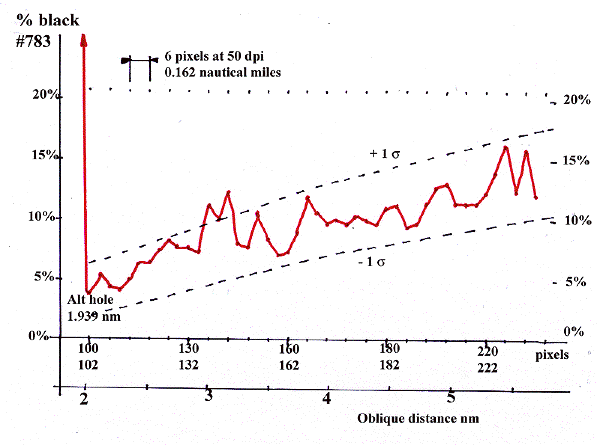Figure 53. Real variation of the average photo darkening along a radius of Figure 52.

It is obvious from Figure 53 that the photo darkening for the ground radar return increases with the radar range, which is exactly what the previous method has predicted. Now let us look at the average darkening at 2.5 nm and 5 nm distances. This average value should be at the middle distance of the two curves +/- 1 standard deviation ( ± 1 σ ), obtained by calculating the square root of the average signal.

• For 5 nm = 12.33 % average darkening.

• For 2.5 nm = 5.33% average darkening.

• The ratio is 12.33 / 5.33 = 2.31.

The theoretical value we should have found is 2.373. The real photometric result shows that the ground radar echo varies like it was predicted from the theory with an accuracy of:

• (2.373 - 2.31) / 2.31 = 2.7%.

This means that our three hidden hypotheses concerning the radar antenna diagram, the tilt-up angle of 50 degrees, and the B-52 “Probable slope” trajectory are correct. In fact, not only are these hypotheses confirmed with an overall accuracy of 2.7%, but other speculations seem to be accurate as well.

For example, the most probable B-52 descent trajectory is the one beginning at FL 200 at the WT fix ending at Final Approach Fix (MM) at an altitude of 3200 feet MSL. The distance traveled by the B-52 during this descent was 28.6 nm and its altitude decreased by 16800 feet; which means an altitude change of 587.4 feet per nautical mile traveled horizontally.

The most probable B-52 altitude during photo 783 was 8865 feet MSL. Since we measured a speed of 254 knots from radarscope photos 782 and 783, the horizontal distance traveled by the B-52 during the 3.0 seconds of each radar photo was 0.212 nm. That is, the most probable B-52 altitude change during each radar photo was 124.34 feet. We can now calculate the B-52 altitude at the end of each radar photo by adding this change to the altitude of the subsequent photo:

• Photo 783: altitude = 8865 feet MSL

• Photo 782: altitude = 8989 feet MSL

• Photo 781: altitude = 9114 feet MSL

• Photo 780: altitude = 9238 feet MSL

• Photo 779: altitude = 9362 feet MSL

• Photo 778: altitude = 9487 feet MSL

• Photo 777: altitude = 9611 feet MSL

• Photo 776: altitude = 9735 feet MSL

• Photo 775: altitude = 9860 feet MSL

• Photo 774: altitude = 9984 feet MSL

• Photo 773: altitude = 10108 feet MSL

• Photo 772: altitude = 10233 feet MSL

• Photo 771: altitude = 10357 feet MSL.

In order to determine the ground altitude of the B-52 we can subtract 1823 feet from the previous values. We find for photo 771 a ground altitude = 10357 - 1823 = 8534 feet, which corresponds to 1.405 nm ground altitude. With a tilt-up angle of 50 degrees for the radar antenna we should have:

• Altitude hole radius 771 = Ground altitude 771 / Cos(50°)

We find the following value:

• Altitude hole radius 771 = 2.19 nm.

The actual altitude hole radius measured on photo 771 is 2.2 nm, which is the same value with an accuracy of 0.65 %. Therefore, the tilt-up angle we used in the analysis report (55.8 degrees) is not the most probable value of 50 degrees. Does this means that we have to recalculate all values depending on the antenna tilt angle? There is more than that to do because this photometric study of the ground echo has presented several other hypotheses, which will be examined later in the second discussion section, entitled, 5-10. Discussion 2: Photometric Study and the Ionized Cloud Surrounding the UFO.

5. The Ionized Cloud Surrounding the UFO ››